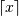# numpy.ceil¶

`numpy.``ceil`(x, /, out=None, *, where=True, casting='same_kind', order='K', dtype=None, subok=True[, signature, extobj]) = <ufunc 'ceil'>

Return the ceiling of the input, element-wise.

The ceil of the scalar x is the smallest integer i, such that i >= x. It is often denoted as.

Parameters: x : array_like Input data. out : ndarray, None, or tuple of ndarray and None, optional A location into which the result is stored. If provided, it must have a shape that the inputs broadcast to. If not provided or None, a freshly-allocated array is returned. A tuple (possible only as a keyword argument) must have length equal to the number of outputs. where : array_like, optional Values of True indicate to calculate the ufunc at that position, values of False indicate to leave the value in the output alone. **kwargs For other keyword-only arguments, see the ufunc docs. y : ndarray or scalar The ceiling of each element in x, with `float` dtype.

Examples

```>>> a = np.array([-1.7, -1.5, -0.2, 0.2, 1.5, 1.7, 2.0])
>>> np.ceil(a)
array([-1., -1., -0.,  1.,  2.,  2.,  2.])
```

numpy.floor

numpy.trunc